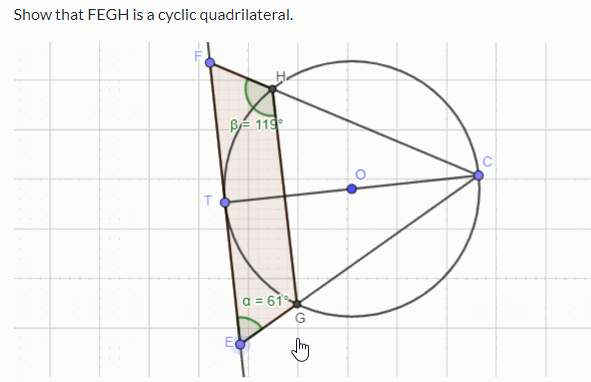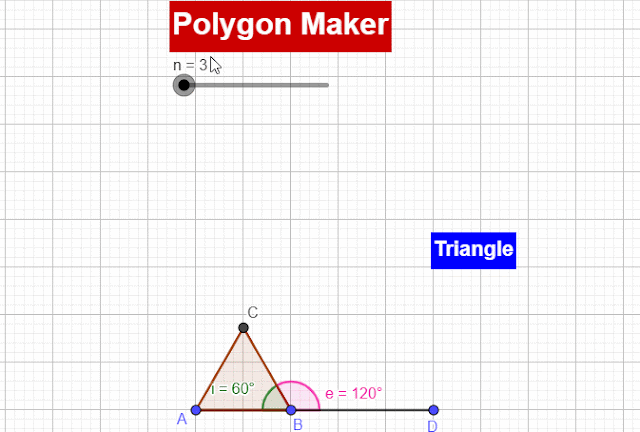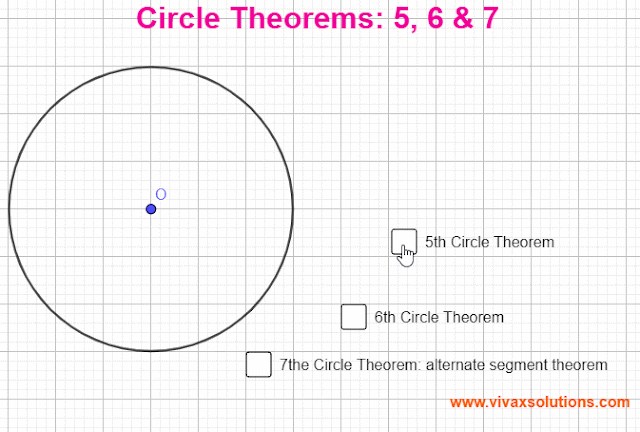## Posts

Showing posts from March 20, 2022

### Circle Theorem Challenges for GCSE, IGCSE, GCE OLChallenge Prove that FEGH in the following simulation is a cyclic quadrilateral. You can use the circle theorems and geometry to prove the above. If you want to practise circle theorems with interactive simulations and learn through worked examples,  please visit this page .

### Polygon Simulator for maths: year 9, GCSE, IGCSE and GCE OLPolygons A closed shape with many sides is a polygon. Sum of Interior Angles If the number of sides is n and the sum of interior angles is T, T = (n-2)180 The table, given below, shows how the sum of interior angles varies with the number of sides. Sum of Exterior Angles The sum of exterior angles is 360 0 , regardless of the number of sides. Polygon No of Sides Sum of Interior Angles Triangle 3 180 0 Square 4 360 0 Pentagon 5 540 0 Hexagon 6 720 0 Heptagon

### Circle Theorems 5, 6 & 7 for GCSE, IGCSE and GCE OLCircle Theorems Circle Theorem 5 The angle between a radius and the tangent at the point of contact is a right angle. Circle Theorem 6 Two tangents drawn from a point to a circle are equal in length. Circle Theorem 7 The angle between a chord and a tangent is equal to the angle in the alternate segment. You can practise the above theorems with the following interactive  simulation: If you want to practise the other circle theorems, please use the following links; they are all interactive: Circle theorems 1, 2 and 3 Circle theorems 4